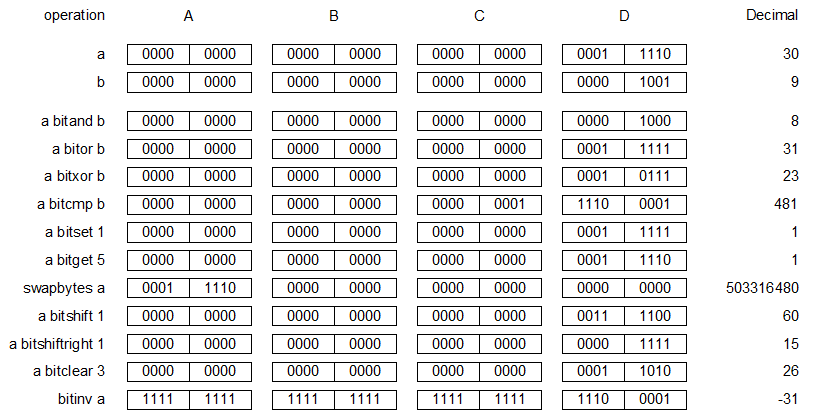﻿ 20-sim webhelp > Language Reference > Operators > Binary > bitor

# bitor

y= a bitor b

## Description

a bitor b performs a bitwise OR between a and b. Both a and b are treated as 32-bit (4-byte) integer values, so an eventual fraction will be ignored.

## Example

In 20-sim, all bitwise function arguments are treated as 32-bit integers. Thus the binary representation of 30 (ignoring leading zeroes) is equal to 11110 as shown in the figure below. Similarly, the binary representation of 9 is 1001. The binary value of 30 bitor 9 is equal to 11111, which corresponds with a decimal value of 31.## Limitations

a and b must be integers.